# Pandas Indexing Examples: Accessing and Setting Values on DataFrames

Last updated:

View all examples on this notebook

## loc example

Use .loc[label_values] to select rows based on their labels.

import pandas as pd

df = pd.DataFrame({
'name':['john','mary','peter','nancy','gary'],
'age':[22,33,27,22,31],
'state':['AK','DC','CA','CA','NY']
})

# select row whose label is 0
df.loc[]

# select rows whose labels are 2 and 3
df.loc[[2,3]]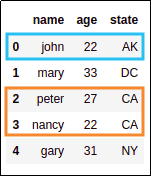Source dataframeselect row whose index label is 0select rows whose index labels are 2 and 3

## loc example with string index

Use .loc[<label_values>] to select rows based on their string labels:

import pandas as pd

# this dataframe uses a custom array as index
df = pd.DataFrame(
index=['john','mary','peter','nancy','gary'],
data={
'age':[22,33,27,22,31],
'state':['AK','DC','CA','CA','NY']
}
)

# select row whose label is 'peter'
df.loc[['peter']]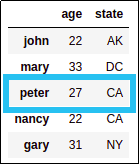Source dataframe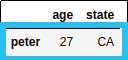selected row whose index label is 'peter'

## iloc example

Use iloc[<element_positions>] to select elements at the given positions (list of ints), no matter what the index is like:

import pandas as pd

df = pd.DataFrame({
'name':['john','mary','peter','nancy','gary'],
'age':[22,33,27,22,31],
'state':['AK','DC','CA','CA','NY']
})

# select row at position 0
df.iloc[]

# select rows at positions 2 through 4
df.iloc[[2,3,4]]Source dataframe with integer index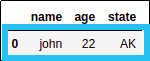selected row at position 0selected rows at positions 2 through 4

Naturally, iloc also works even if you have a string index:

import pandas as pd

# this dataframe uses a custom array as index
df = pd.DataFrame(
index=['john','mary','peter','nancy','gary'],
data={
'age':[22,33,27,22,31],
'state':['AK','DC','CA','CA','NY']
}
)

# select row at position 0
df.iloc[]

# select rows at positions 2 through 4
df.iloc[[2,3,4]]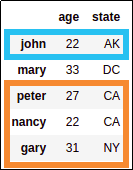Source dataframe with string index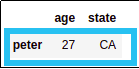selected row at position 0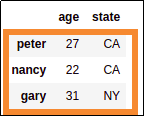selected rows at positions 2 through 4

## loc vs iloc

• loc and iloc behave the same whenever your dataframe has an integer index starting at 0

loc iloc
Select by element labelSelect by element position
Can be used for setting
individual values to cells
Cannot be used for setting
individual values to cells

## set value to individual cell

For setting an individual value, you must use .loc.

In other words, assign a value to a specific cell in a dataframe.

import pandas as pd

df = pd.DataFrame({
'name':['john','mary','peter','nancy','gary'],
'age':[22,33,27,22,31],
'state':['AK','DC','CA','CA','NY']
})

# set individual value
df.loc[0,'name'] = 'bartholomew'

# set individual value once more
df.loc[3, 'age'] = 39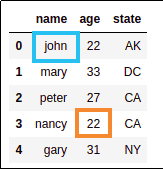BEFORE: Source dataframe with
original values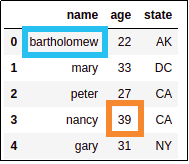AFTER: changed john's name
to bartholomew and changed
nancy's age to 39

## fix SettingWithCopyWarningAnnoying, right?

SettingWithCopyWarning happens when you try to assign data to a dataframe that was derived from another dataframe.

One quick way to fix it is to create a copy of the source dataframe before operating.

For example: from a source dataframe, selecting only people older than 30:

import pandas as pd

# source dataframe
df = pd.DataFrame({
'name':['john','mary','peter','nancy','gary'],
'age':[22,33,27,22,31],
'state':['AK','DC','CA','CA','NY']
})

• BAD (operating on the source dataframe directly)

# create a derived dataset for people over 30 years of age
df_over_30_years = df[df['age']>30]

df_over_30_years['new_column'] = 'some_value'
#>>> SettingWithCopyWarning:
#>>> A value is trying to be set on a copy of a slice from a DataFrame.
#>>> Try using .loc[row_indexer,col_indexer] = value instead

• GOOD: (call copy() on the source dataframe first, and then add a new column)

# by using .copy(), you're not operating on the source dataframe anymore!
df_over_30_years = df.copy()[df['age']>30]

# no error now
df_over_30_years['new_column'] = 'some_value'